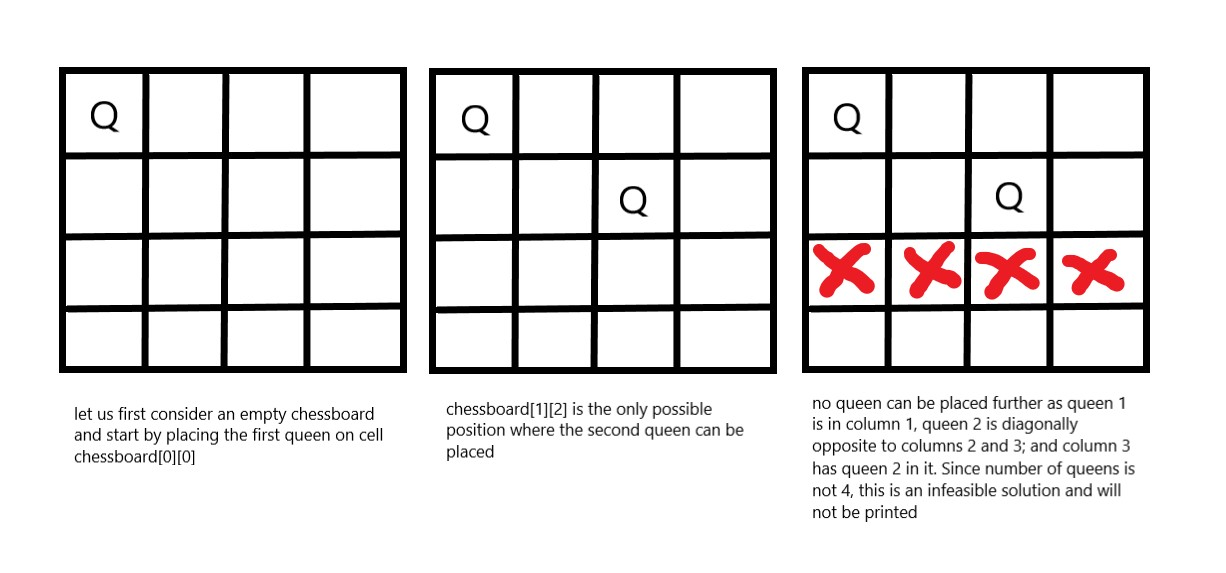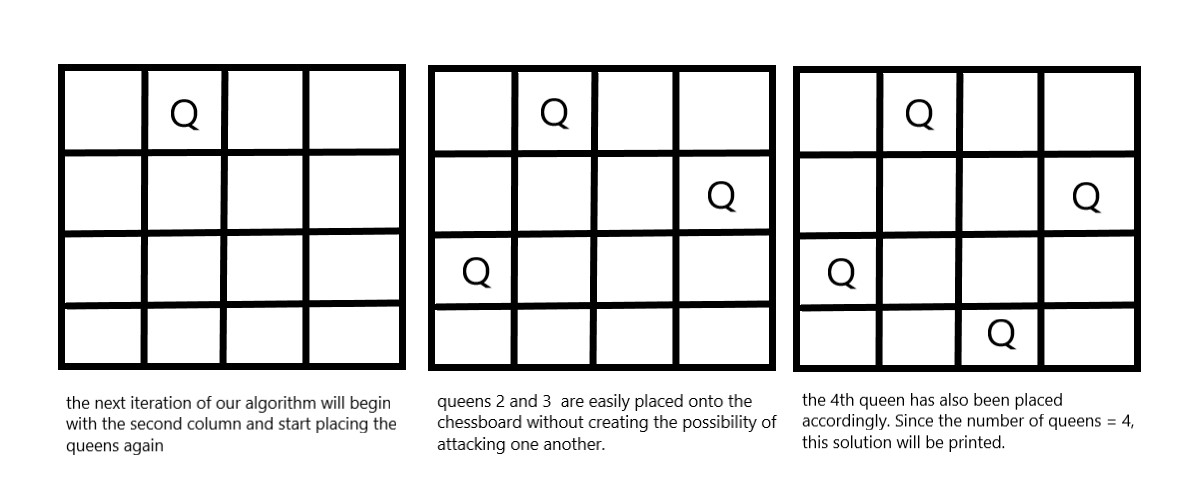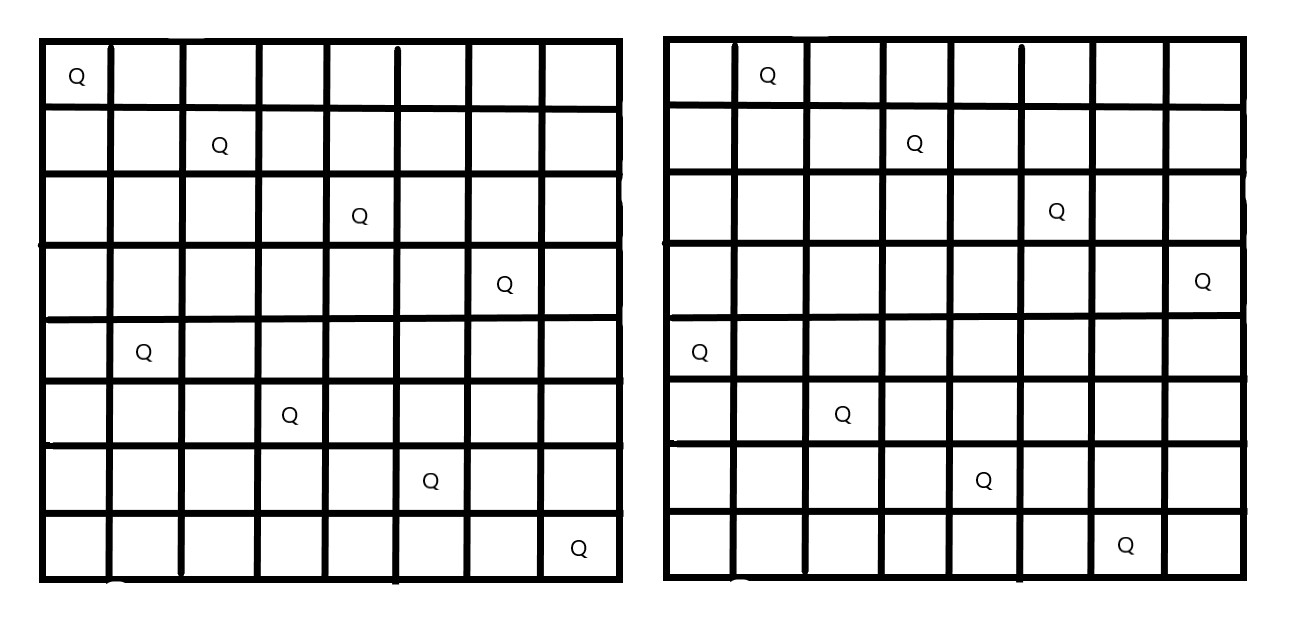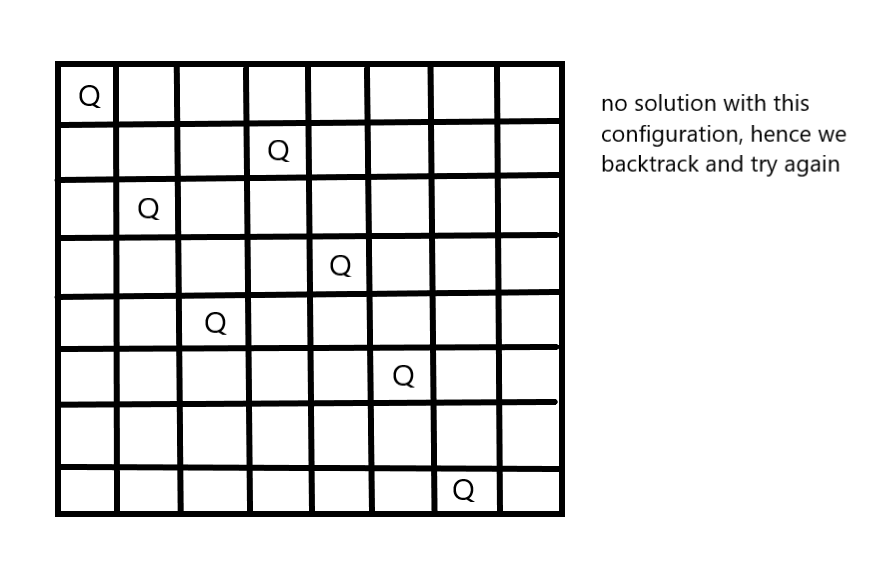×

Search anything:

# 8 Queens Problem using Backtracking

#### Algorithms backtrackingGet this book -> Problems on Array: For Interviews and Competitive Programming

Reading time: 30 minutes | Coding time: 10 minutes

You are given an 8x8 chessboard, find a way to place 8 queens such that no queen can attack any other queen on the chessboard. A queen can only be attacked if it lies on the same row, or same column, or the same diagonal of any other queen. Print all the possible configurations.

To solve this problem, we will make use of the Backtracking algorithm. The backtracking algorithm, in general checks all possible configurations and test whether the required result is obtained or not. For thr given problem, we will explore all possible positions the queens can be relatively placed at. The solution will be correct when the number of placed queens = 8.

The time complexity of this approach is O(N!).

Input Format - the number 8, which does not need to be read, but we will take an input number for the sake of generalization of the algorithm to an NxN chessboard.

Output Format - all matrices that constitute the possible solutions will contain the numbers 0(for empty cell) and 1(for a cell where queen is placed). Hence, the output is a set of binary matrices.

Visualisation from a 4x4 chessboard solution :

In this configuration, we place 2 queens in the first iteration and see that checking by placing further queens is not required as we will not get a solution in this path. Note that in this configuration, all places in the third rows can be attacked.As the above combination was not possible, we will go back and go for the next iteration. This means we will change the position of the second queen.In this, we found a solution.

Now let's take a look at the backtracking algorithm and see how it works:

The idea is to place the queens one after the other in columns, and check if previously placed queens cannot attack the current queen we're about to place.

If we find such a row, we return true and put the row and column as part of the solution matrix. If such a column does not exist, we return false and backtrack*

## Pseudocode

``````START
1. begin from the leftmost column
2. if all the queens are placed,
return true/ print configuration
3. check for all rows in the current column
a) if queen placed safely, mark row and column; and
recursively check if we approach in the current
configuration, do we obtain a solution or not
b) if placing yields a solution, return true
c) if placing does not yield a solution, unmark and
try other rows
4. if all rows tried and solution not obtained, return
false and backtrack
END
``````

## Implementation

Implementaion of the above backtracking algorithm :

``````#include <bits/stdc++.h>

using namespace std;

int board; // you can pick any matrix size you want

bool isPossible(int n,int row,int col){  // check whether
// placing queen possible or not

// Same Column
for(int i=row-1;i>=0;i--){
if(board[i][col] == 1){
return false;
}
}

//Upper Left Diagonal
for(int i=row-1,j=col-1;i>=0 && j>=0 ; i--,j--){
if(board[i][j] ==1){
return false;
}
}

// Upper Right Diagonal
for(int i=row-1,j=col+1;i>=0 && j<n ; i--,j++){
if(board[i][j] == 1){
return false;
}
}

return true;
}
void nQueenHelper(int n,int row){
if(row==n){
// We have reached some solution.
// Print the board matrix
// return

for(int i=0;i<n;i++){
for(int j=0;j<n;j++){
cout << board[i][j] << " ";
}
}
cout<<endl;
return;

}

// Place at all possible positions and move to smaller problem
for(int j=0;j<n;j++){

if(isPossible(n,row,j)){  // if no attack, proceed
board[row][j] = 1;      // mark row, column with 1
nQueenHelper(n,row+1);  // call function to continue
// further
}

board[row][j] = 0;      // unmark to backtrack
}
return;

}
void placeNQueens(int n){

memset(board,0,8*8*sizeof(int)); // allocate 8*8 memory
// and initialize all
// cells with zeroes

nQueenHelper(n,0);     // call the backtracking function
// and print solutions
}

int main(){

int n;
cin>>n; // could use a default 8 as well

placeNQueens(n);
return 0;
}
``````

Output ( for n = 4): 1 indicates placement of queens

`````` 0  0  1  0
1  0  0  0
0  0  0  1
0  1  0  0
``````

Explanation of the above code solution:These are two possible solutions from the entire solution set for the 8 queen problem.

``````main()
{
call placeNQueens(8),

placeNQueens(){

call nQueenHelper(8,0){  row = 0

if(row==n) // won't execute as 0 != 8

for(int j=0; j<8; j++){
{
if(isPossible==true)
{  board = 1  // board[row] = 1

call nQueenHelper(8,row+1)  // recur for all rows further

print matrix when row = 8 if solution obtained
and (row==n) condition is met

}
board = 0  // backtrack and try for
// different configurations
}
}

}
}
``````

for example, the following configuration won't be displayed## Time Complexity Analysis

1. the isPossible method takes O(n) time
2. for each invocation of loop in nQueenHelper, it runs for O(n) time
3. the isPossible condition is present in the loop and also calls nQueenHelper which is recursive

adding this up, the recurrence relation is:

T(n) = O(n^2) + n * T(n-1)

solving the above recurrence by iteration or recursion tree,
the time complexity of the nQueen problem is = O(N!)

## Question :-

You are given an NxN maze with a rat placed at (0,0). Find and print all the paths that the rat can follow to reach its destination i.e (N-1,N-1). The rat can move in all four directions (left,right,up,down).
Value of every cell will be either 0 or 1. 0 represents a blocked cell, the rat cannot move through it, though 1 is an unblocked cell.
Solve the problem using backtracking algorithm,

Input - an integer N and a binary maze of size NxN, showing blocked and
unblocked cells.
Output - all possible path matrices the rat can travel from (0,0) to (N-1,N-1).